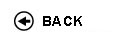Politecnico di Torino
Academic Year 2017/18
05KXWJM, 05KXWLI
Physics II
1st degree and Bachelor-level of the Bologna process in Mechanical Engineering - Torino
1st degree and Bachelor-level of the Bologna process in Automotive Engineering - Torino
 Teacher Status SSD Les Ex Lab Tut Years teaching Tagliaferro AlbertoAC FIS/03 39 21 0 0 5
 SSD CFU Activities Area context FIS/01FIS/03 33 A - Di baseA - Di base Fisica e chimicaFisica e chimica
 Subject fundamentals Aim of the course (1st semester, 2nd year) is to provide the students of Electronic, Telecommunications, Computer and Physical Engineering with the theoretical concepts to be used in all courses of the following semesters. This is therefore a pivotal course for the ensuing career. Subjects of basic physics such as electromagnetism, the Maxwell's equations and the physical optics are treated together with the crisis of classical mechanics and the transition to the fundaments of modern physics. Expected learning outcomes - Knowledge of electrostatics in dielectrics media and of magnetostatics. - Knowledge of basic principles of time-dependent electric and magnetic fields. - Knowledge of Maxwell's equations. - Knowledge of wave optics as a consequence of Maxwell's equations. - Knowledge of wave optics laws and of properties of electromagnetic waves. - Preliminary knowledge of the wave-particle dualism. - Ability to apply the acquired knowledge to solve elementary problems. Prerequisites / Assumed knowledge - Basic physics (mechanics, thermodynamics, basic electrostatics) - Basic mathematics and geometry Contents ELECTROSTATICS (1 CFU) Static electric field in matter: conductors. Capacitance and capacitors. Energy density of the electric field. Dielectric materials: electrical polarization. Electric current. Conduction. Current intensity and current density. Direct current (DC). Resistance. Ohm’s law. Resistivity and conductivity. Electric power. Joule effect. MAGNETOSTATICS (1,5 CFU) Magnetic field and magnetic induction. Second Maxwell’s equation. Force on a charge moving in a magnetic field: Lorentz’s force. Magnetic force on a current-carrying conductor. Sources of magnetic field. Field of a straight current-carrying conductor: Laplace’s law, and its applications. Magnetic field of a circular current loop. Magnetic dipole. Torque on, and potential energy of, a magnetic dipole in a magnetic field. Forces between parallel currents. Ampčre’s law and its applications. Magnetic fields in matter: diamagnetism, paramagnetism and ferromagnetism. TIME-DEPENDENT ELECTRIC AND MAGNETIC FIELDS (2 CFU) Faraday – Henry – Lenz law of electromagnetic induction and its applications. Third Maxwell’s equation. Inductance and self-inductance. Energy in an R-L circuit. Energy density of the magnetic field. Ampčre-Maxwell law: fourth Maxwell’s equation. ELECTROMAGNETIC WAVES (1 CFU) Wave equation for electric and magnetic field. General characteristics of a wave. Electromagnetic waves. Propagation and attenuation of the electromagnetic waves in conductors and dielectrics. Wave optics. Interference, electromagnetic waves interference and its applications. Diffraction: the basic principles. Fraunhofer’s theory of a double slit interference and of a single slit diffraction. Polarization of light: the basic principles. INTRODUCTION TO MODERN PHYSICS (0,5 CFU) Photons. Waves and particles. De Broglie. Delivery modes Class exercises concern with simple problem solving activities, in strict correlation with the previous theoretical lectures. In some cases scientific calculators (students' personal property) may be required. Texts, readings, handouts and other learning resources • "Physics for Scientists and Engineers with Modern Physics" FISHBANE, GASIOROWICZ, THORNTON, Ed. Pearson, Prentice Hall Learning material distributed by teacher. Assessment and grading criteria The exam is organized in two parts: written and oral. The written part consists of two quizzes and two exercises. A threshold mark is required to get through to the oral. The oral part consists in a single question on one of the subjects of the syllabus. The marks are handled as follows: 1) each of the written parts awards a maximum of 10.5 points for a maximum total mark for the written part of 21 2) the oral part awards a maximum of 11 points 3) the final mark is the aritmetic addition of the written and the oral marks 4) each of the following situations lead to exam failure: - mark for at least one of the written parts < 3 - total mark for the written part < 10 - mark for the oral part < 6 Programma definitivo per l'A.A.2017/18© Politecnico di Torino
Corso Duca degli Abruzzi, 24 - 10129 Torino, ITALY